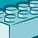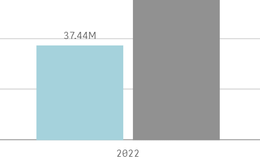# New to Qlik Sense

If you’re new to Qlik Sense, start with this Discussion Board and get up-to-speed quickly.

Announcements
QlikWorld 2023, a live, in-person thrill ride. Save \$300 before February 6: REGISTER NOW!
cancel
Showing results for
Did you mean:Contributor III

## How to calculate Dimension for Top 20 Suppliers by Spend and per Year in Qlik Sense?

Hi Everyone,

I am trying to create a dimension that calculates the spending of the top 20 suppliers by spend and per year.

My current formula is the following:

``=sum({<[Supplier ] = {"=Aggr(Rank(Sum([Spend]))<=20, [Supplier]) "}>} [Spend])``

I have data between 2019 to 2022 YTD. I use this dimension in a bar chart in which the X-axis shows the Years.

When I select one year, the dimension is calculating the correct figure. However, if I don't select any year, the formula is currently checking the top 20 suppliers for all years and then it checks the spending of these suppliers in each year.

I need that the total spend per year is the same regardless if I select one year or not.

Labels (1)
• ### expression

2 Solutions

Accepted SolutionsMVP

Hi @Goncalo

The expression I gave the first time out required that the Supplier was one of the dimensions, without that you will need to add it into the aggr.

So, the expression is now:

``=sum(aggr(if(rank(sum(Spend)) <= 20, sum(Spend), null()), Year, Supplier))``

This screengrab shows it working, with the 2020 value being the same regardless of selection.Test data generated with:

``````Random:
chr(65 + floor(rand()*4)) & chr(65 + floor(rand()*26)) as Supplier,
2019 + floor(rand()*4) as Year,
ceil(rand()*1000) as Spend
AUTOGENERATE(500)
;``````

The whole app is attached should you want to take a look.

Hope that helps.

SteveMVP

Hi @Goncalo

I think the way you approach it for months would have to be different, as you are working out the top 20 at a different level of dimensionality from what the chart is at.

I think that this code should do it:

``````=if(GetPossibleCount(Year) > 1,
sum(aggr(if(rank(sum(Spend)) <= 20, sum(Spend), null()), Year, Supplier)),
sum({<Supplier={'\$(=concat(TOTAL aggr(if(rank(sum(Spend)) <= 20, Supplier, null()), Supplier), chr(39) & ',' & chr(39)))'}>} Spend))``````

You will recognize the old expression in there (in italics), the other expression gets the list of suppliers outside of the expression and then injects it in using Set Analysis.

The loaded script to test this one is:

``````Random:
*,
Year(Month) as Year
;
chr(65 + floor(rand()*4)) & chr(65 + floor(rand()*26)) as Supplier,
ceil(rand()*1000) as Spend
AUTOGENERATE(2000)
;``````

And again the app is attached.

Cheers,
Steve

17 RepliesMVP

Hi @Goncalo

One way of achieving this is:

``=if(rank(sum(Spend)) <= 20, sum(Spend), null())``

You will need to have the Supplier as a dimension and stack them for this approach to work.

The one drawback I can see is if there are two suppliers with the same spend in the year at position 20 then both could get included.

Hope that moves you forward.

SteveContributor III
Author

Thanks for the feedback!

The current formula I am using already groups spend by supplier. The issue is that if I don't select a specific year, it will look for the spend by supplier for all years and then looks for the top 20 in this timeframe.

However, if I select one year the value changes as it is only looking for the top 20 for that year.

Chart data without a year selected:Chart data with 2022 selected:I would like to see the 37.44M with or without a year selected.

I am guessing I would need to aggregate by year before checking the top 20 suppliers, but it did not work in the iterations I tried.

Any suggestions?MVP

Hi @Goncalo

The expression I gave the first time out required that the Supplier was one of the dimensions, without that you will need to add it into the aggr.

So, the expression is now:

``=sum(aggr(if(rank(sum(Spend)) <= 20, sum(Spend), null()), Year, Supplier))``

This screengrab shows it working, with the 2020 value being the same regardless of selection.Test data generated with:

``````Random:
chr(65 + floor(rand()*4)) & chr(65 + floor(rand()*26)) as Supplier,
2019 + floor(rand()*4) as Year,
ceil(rand()*1000) as Spend
AUTOGENERATE(500)
;``````

The whole app is attached should you want to take a look.

Hope that helps.

SteveContributor III
Author

Thanks Steve! It is working perfectly!MVP

Thanks for confirming @Goncalo , and thanks for marking as the solution - this really helps other Community members.

Cheers,
SteveContributor III
Author

Hi @stevedark I have one follow-up question on this, perhaps it is a variation of your solution.

I added a drilldown with Year and Month, but the values for the months are not showing the correct figures, i.e., the value per year is correct, but once I click to see the spend by month, the values do not match the actual month spend figures.

Thanks!MVP

Hi @Goncalo

I think the way you approach it for months would have to be different, as you are working out the top 20 at a different level of dimensionality from what the chart is at.

I think that this code should do it:

``````=if(GetPossibleCount(Year) > 1,
sum(aggr(if(rank(sum(Spend)) <= 20, sum(Spend), null()), Year, Supplier)),
sum({<Supplier={'\$(=concat(TOTAL aggr(if(rank(sum(Spend)) <= 20, Supplier, null()), Supplier), chr(39) & ',' & chr(39)))'}>} Spend))``````

You will recognize the old expression in there (in italics), the other expression gets the list of suppliers outside of the expression and then injects it in using Set Analysis.

The loaded script to test this one is:

``````Random:
*,
Year(Month) as Year
;
chr(65 + floor(rand()*4)) & chr(65 + floor(rand()*26)) as Supplier,
ceil(rand()*1000) as Spend
AUTOGENERATE(2000)
;``````

And again the app is attached.

Cheers,
SteveContributor III
Author

Hi @stevedark!

Again, many thanks for your help! The code above was not working for months right away. I believe it may be due to the way the data model I'm using is set up, which I was not able to explain properly.

However, by looking at your approach I repeated the first part of the if in the second part and replaced the Year by Month, and it worked!

``````=if(GetPossibleCount(Year) > 1,
sum(aggr(if(rank(sum(Spend)) <= 20, sum(Spend), null()), Year, Supplier)),
sum(aggr(if(rank(sum(Spend)) <= 20, sum(Spend), null()), Month, Supplier)))``````

Again, thanks for your support and patience, marked as a solution.

Cheers,

GoncaloMVP

You might be looking at the top 20 in each month though, rather than the top 20 in the year split across months, or is that what you are after?

Thanks,

SteveTags
Community Browser